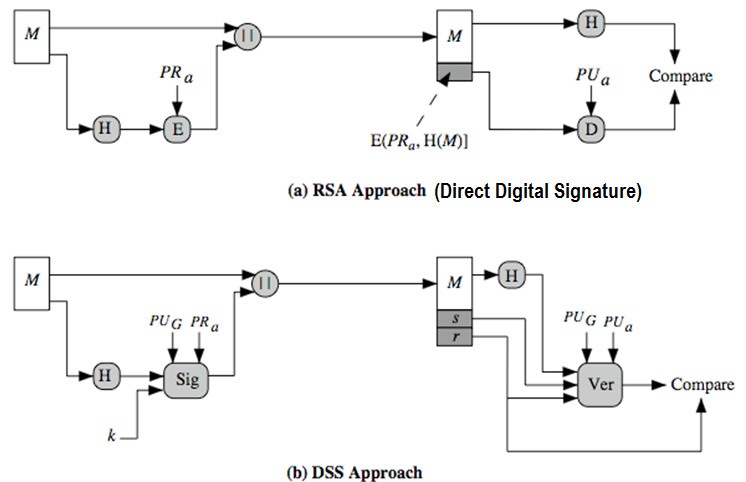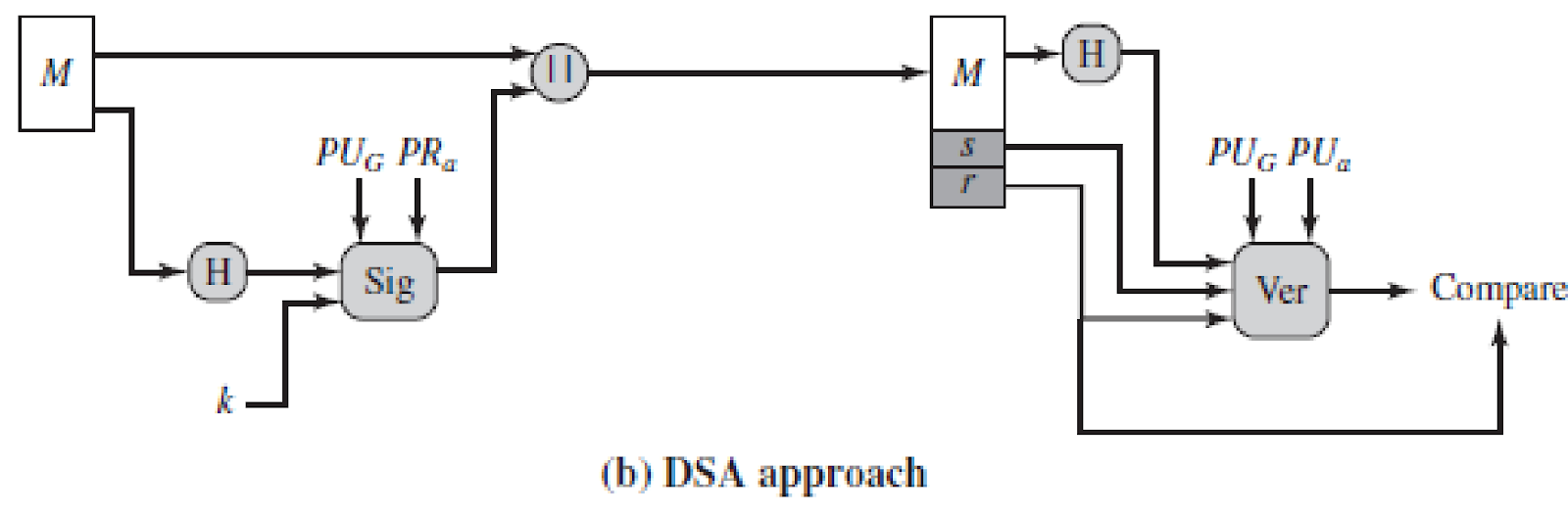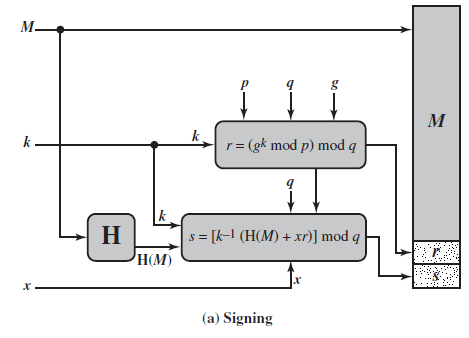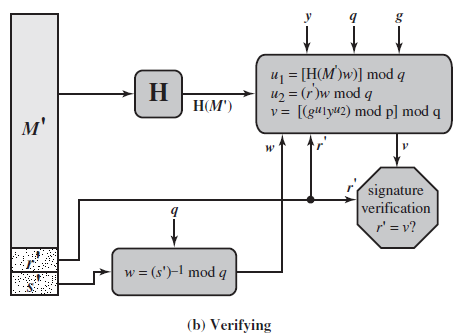# Explain various digital signature schemes with suitable diagram?

A digital signature is a mathematical scheme for verifying the authenticity of digital messages or documents. A valid digital signature, where the prerequisites are satisfied, gives a recipient very strong reason to believe that the message was created by a known sender (authentication), and that the message was not altered in transit (integrity). Digital signatures are a standard element of most cryptographic protocol suites, and are commonly used for software distribution, financial transactions, contract management software, and in other cases where it is important to detect forgery or tampering.

Digital signatures are the public-key primitives of message authentication. In the physical world, it is common to use handwritten signatures on handwritten or typed messages. They are used to bind signatory to the message.

Similarly, a digital signature is a technique that binds a person/entity to the digital data. This binding can be independently verified by receiver as well as any third party.

Digital signature is a cryptographic value that is calculated from the data and a secret key known only by the signer.

In real world, the receiver of message needs assurance that the message belongs to the sender and he should not be able to repudiate the origination of that message. This requirement is very crucial in business applications, since likelihood of a dispute over exchanged data is very high.

## Model of Digital Signature

As mentioned earlier, the digital signature scheme is based on public key cryptography. The model of digital signature scheme is depicted in the following illustration −

The following points explain the entire process in detail −

• Each person adopting this scheme has a public-private key pair.
• Generally, the key pairs used for encryption/decryption and signing/verifying are different. The private key used for signing is referred to as the signature key and the public key as the verification key.
• Signer feeds data to the hash function and generates hash of data.
• Hash value and signature key are then fed to the signature algorithm which produces the digital signature on given hash. Signature is appended to the data and then both are sent to the verifier.
• Verifier feeds the digital signature and the verification key into the verification algorithm. The verification algorithm gives some value as output.
• Verifier also runs same hash function on received data to generate hash value.
• For verification, this hash value and output of verification algorithm are compared. Based on the comparison result, verifier decides whether the digital signature is valid.
• Since digital signature is created by ‘private’ key of signer and no one else can have this key; the signer cannot repudiate signing the data in future.

It should be noticed that instead of signing data directly by signing algorithm, usually a hash of data is created. Since the hash of data is a unique representation of data, it is sufficient to sign the hash in place of data. The most important reason of using hash instead of data directly for signing is efficiency of the scheme.

Let us assume RSA is used as the signing algorithm. As discussed in public key encryption chapter, the encryption/signing process using RSA involves modular exponentiation.

Signing large data through modular exponentiation is computationally expensive and time consuming. The hash of the data is a relatively small digest of the data, hence signing a hash is more efficient than signing the entire data.

## Importance of Digital Signature

Out of all cryptographic primitives, the digital signature using public key cryptography is considered as very important and useful tool to achieve information security.

Apart from ability to provide non-repudiation of message, the digital signature also provides message authentication and data integrity. Let us briefly see how this is achieved by the digital signature −

• Message authentication − When the verifier validates the digital signature using public key of a sender, he is assured that signature has been created only by sender who possess the corresponding secret private key and no one else.
• Data Integrity − In case an attacker has access to the data and modifies it, the digital signature verification at receiver end fails. The hash of modified data and the output provided by the verification algorithm will not match. Hence, receiver can safely deny the message assuming that data integrity has been breached.
• Non-repudiation − Since it is assumed that only the signer has the knowledge of the signature key, he can only create unique signature on a given data. Thus the receiver can present data and the digital signature to a third party as evidence if any dispute arises in the future.

By adding public-key encryption to digital signature scheme, we can create a cryptosystem that can provide the four essential elements of security namely − Privacy, Authentication, Integrity, and Non-repudiation.

## Encryption with Digital Signature

In many digital communications, it is desirable to exchange an encrypted messages than plaintext to achieve confidentiality. In public key encryption scheme, a public (encryption) key of sender is available in open domain, and hence anyone can spoof his identity and send any encrypted message to the receiver.

This makes it essential for users employing PKC for encryption to seek digital signatures along with encrypted data to be assured of message authentication and non-repudiation.

This can archived by combining digital signatures with encryption scheme. Let us briefly discuss how to achieve this requirement. There are two possibilities, sign-then-encrypt and encrypt-then-sign.

However, the crypto system based on sign-then-encrypt can be exploited by receiver to spoof identity of sender and sent that data to third party. Hence, this method is not preferred. The process of encrypt-then-sign is more reliable and widely adopted. This is depicted in the following illustration −

The receiver after receiving the encrypted data and signature on it, first verifies the signature using sender’s public key. After ensuring the validity of the signature, he then retrieves the data through decryption using his private key

DSS vs RSA SignaturesPRa – private key of sender

PUa - public key of sender

k- a random number generated for this particular signature.

PUG- global public key known to a group of communicating nodes

Signature consists of two parts- s & r

Stallings Figure 13.3 contrasts the DSS approach for generating digital signatures to that used with RSA. In the RSA approach, the message to be signed is input to a hash function that produces a secure hash code of fixed length. This hash code is then encrypted using the sender's private key to form the signature. Both the message and the signature are then transmitted. The recipient takes the message and produces a hash code. The recipient also decrypts the signature using the sender's public key. If the calculated hash code matches the decrypted signature, the signature is accepted as valid. Because only the sender knows the private key, only the sender could have produced a valid signature.  The DSS approach also makes use of a hash function. The hash code is provided as input to a signature function along with a random number k generated for this particular signature. The signature function also depends on the sender's private key (PR a) and a set of parameters known to a group of communicating principals. We can consider this set to constitute a global public key (PUG).   The result is a signature consisting of two components, labeled s and r.  At the receiving end, the hash code of the incoming message is generated. This plus the signature is input to a verification function. The verification function also depends on the global public key as well as the sender's public key (PUa), which is paired with the sender's private key. The output of the verification function is a value that is equal to the signature component r if the signature is valid. The signature function is such that only the sender, with knowledge of the private key, could have produced the valid signature.

Digital Signature Algorithm (DSA)

• creates a 320 bit signature
• smaller and faster than RSA
• a digital signature scheme only
• it cannot be used for encryption or key exchangeThe DSA is based on the difficulty of computing discrete logarithms (see Chapter 8) and is based on schemes originally presented by ElGamal [ELGA85] and Schnorr [SCHN91]. The DSA signature scheme has advantages, being both smaller (320 vs 1024bit) and faster (much of the computation is done modulo a 160 bit number), over RSA. Unlike RSA, it cannot be used for encryption or key exchange. Nevertheless, it is a public-key technique

• The DSA approach also makes use of a hash function
• The hash code is provided as input to a signature function along with a random number k  generated for this particular signature.
• The signature function also depends on the sender’s private key (PRa) and a set of parameters (PUG) known to a group of communicating nodes. PUG can be considered as a global public key.
• The result is a signature consisting of two components, labeled s  and r.

The DSA uses an algorithm that is designed to provide only the digital signature

function. Unlike RSA, it cannot be used for encryption or key exchange.

Nevertheless, it is a public-key technique.

Figure 13.2 contrasts the DSA approach for generating digital signatures to

that used with RSA. In the RSA approach, the message to be signed is input to a

hash function that produces a secure hash code of fixed length. This hash code is

then encrypted using the sender’s private key to form the signature. Both the message

and the signature are then transmitted. The recipient takes the message and

produces a hash code. The recipient also decrypts the signature using the sender’s

public key. If the calculated hash code matches the decrypted signature, the signature

is accepted as valid. Because only the sender knows the private key, only the

sender could have produced a valid signature.

The DSA approach also makes use of a hash function. The hash code is provided

as input to a signature function along with a random number k  generated

for this particular signature. The signature function also depends on the sender’s

private key (PRa ) and a set of parameters known to a group of communicating principals.

We can consider this set to constitute a global public key (PUG ).  The result

is a signature consisting of two components, labeled s  and r .

At the receiving end, the hash code of the incoming message is generated. This

plus the signature is input to a verification function. The verification function also

depends on the global public key as well as the sender’s public key (PUa ), which

is paired with the sender’s private key. The output of the verification function is a

value that is equal to the signature component r  if the signature is valid. The signature

function is such that only the sender, with knowledge of the private key, could

have produced the valid signature.

DSA Key Generation

• have shared global public key values (p,q,g):
• choose a large prime p with 2L-1 < p < 2L
• where L= 512 to 1024 bits and is a multiple of 64
• choose 160-bit prime number  q
• such that q is a 160 bit prime divisor of (p-1)
• choose g = h(p-1)/q
• where  1 < h < p-1 and h(p-1)/q mod p > 1
• users choose private key & compute public key:
• choose random private key:  x<q
• compute public key: y = gx mod p
• Choose user’s per message secret number,  k
• Such the k is a pseudo-random number 0< k<q

DSA typically uses a common set of global parameters (p,q,g) for a community of clients, as shown. A 160-bit prime number q is chosen. Next, a prime number p is selected with a length between 512 and 1024 bits such that q divides (p – 1). Finally, g is chosen to be of the form h(p–1)/q mod p where h is an integer between 1 and (p – 1) with the restriction that g must be greater than 1. Thus, the global public key components of DSA have the same for as in the Schnorr signature scheme.

Then each DSA uses chooses a random private key x, and computes their public key as shown. The calculation of the public key y given x is relatively straightforward. However, given the public key y, it is computationally infeasible to determine x, which is the discrete logarithm of y to base g, mod p.

DSA  Signature Creation

• to sign a message M the sender:
• generates a random signature key k,k<q
• k is used only once, never reused
• then computes signature pair

r = (gk mod p)mod q

s = [k-1(H(M)+ x r)] mod q

• sends signature (r,s) with message MM = message to be signed

H(M) = hash of M using SHA-1

X = private key

To create a signature, a user calculates two quantities, r and s, that are functions of the public key components (p,q,g), the user’s private key (x), the hash code of the message H(M), and an additional integer k that should be generated randomly or pseudo-randomly and be unique for each signing. This is similar to ElGamal signatures, with the use of a per message temporary signature key k, but doing calculations first mod p, then mod q to reduce the size of the result. The signature (r,s) is then sent with the message to the recipient. Note that computing r only involves calculation mod p and does not depend on message, hence can be done in advance. Similarly with randomly choosing k’s and computing their inverses.

DSA Signature Verificationy = PUa, public key of sender

M’, r’, s’ = received versions of M, r, s

• having received M & signature (r,s)
• to verify a signature, recipient computes:

w = s’-1 mod q

u1= [H(M’)w ]mod q

u2= (r’w)mod q

v = [(gu1 yu2)mod p ]mod q

• if v=r’,  then signature is verified

At the receiving end, verification is performed using the formulas shown. The receiver generates a quantity v that is a function of the public key components, the sender’s public key, and the hash of the incoming message. If this quantity matches the r component of the signature, then the signature is validated. Note that the difficulty of computing discrete logs is why it is infeasible for an opponent to recover k from r, or x from s. Note also that nearly all the calculations are mod q, and hence are much faster save for the last step.

The structure of this function is such that the receiver can recover r using the incoming message and signature, the public key of the user, and the global public key. It is certainly not obvious that such a scheme would work. A proof is provided in Stallings appendix K.

DSA Signing and Verifying

• Given the difficulty of taking discrete logarithms, it is infeasible for an opponent to recover k from r or to recover x from s.
• The only computationally demanding task in signature generation is the exponential calculation gk mod p. Because this value does not depend on the message to be signed, it can be computed ahead of time.
• a user could pre-calculate a number of values of r to be used to sign documents as needed.
• other demanding task is the determination of a multiplicative inverse, k-1. Again, a number of these values can be pre-calculated.

Figure 13.4 depicts the functions of signing and verifying.

The structure of the algorithm, as revealed in Figure 13.4, is quite interesting.

Note that the test at the end is on the value r , which does not depend on the message

at all. Instead, r  is a function of k  and the three global public-key components. The

multiplicative inverse of k  (mod q ) is passed to a function that also has as inputs

the message hash code and the user’s private key. The structure of this function is

such that the receiver can recover r  using the incoming message and signature, the

public key of the user, and the global public key. It is certainly not obvious from

Figure 13.3 or Figure 13.4 that such a scheme would work. A proof is provided in

Appendix K.

Comparison of DSS and RSA

Difference:

• DSS provides digital signatures. But does not provide key exchange and encryption. RSA provides digital signatures, encryption and key exchange.

Similarity:

• Both DSS and RSA are based on public (Asymmetric) key technique (Private and public keys are used. Private key of sender on sender's side and public key of sender on the receiver side)

RSA approach:

• The message is input to a hash function.
• The hash value is then encrypted using the sender's private key.
• This encrypted content serves as the signature. It is attached  with the original message and transmitted.
• The receiver generates a hash code and decrypts the signature component using the sender's public key.
• If the generated hash code and the decrypted value matches, the message is authenticated.

DSS approach:

• The hash code is generated from the message
• This hash code, along with a random number generated k is given as input to the signature function.
• The sender's private key and a global public key is also given as input to the signature function.
• The output of this signature function is attached with the original message and sent.

During reception, the hash function is generated.

• Along with this, the signature is sent to a verification function.
• The verification function depends on the sender's public key and the global public key.
• This is compared with the signature component of the incoming message.
• If they both match, the incoming message is authenticated.